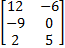Matrices can only be added if they are the same classification,meaning they have the same number of rows and the same number of columns. To add or subtract matrices.... 1)Make sure they have the same number of rows and columns.(If not, you cannot add them.) 2)Add or subtract each set of corresponding entries. For example: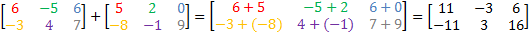You don't necessarily have to list the intermediate step in which you write out each pair of addition, but you do always have to be very careful with your negatives. When you are subtracting matrices, you need to be extra careful with your negatives, and you should probably write out each set of entries. For example,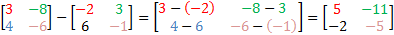Practice: Add or subtract the following matrices. 1)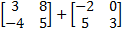2)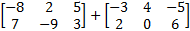3)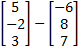4)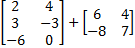5)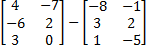Answers:1)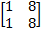2)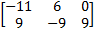3)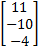4) cannot add: not same classification5)